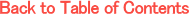Adv. Math. Econ. Volume 6, pp.39-53 (2004)

The compactness of Pr(K)

Dionysius Glycopantis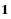, Allan Muir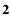Department of Economics, City University, Northampton Square, London EC1V 0HB, UKDepartment of Mathematics, City University, Northampton Square, London EC1V 0HB, UK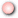PDF styleDVI style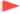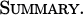We prove the compactness of Pr(K), the set of Borel probability measures on a compactum K endowed with the weak* topology, without embedding this set in rca(K), the space of regular, countably-additive, signed measures with their finite total variation as norm. Pr(K) can be extended to a convex, Hausdorff, linear topological space. Then Glicksberg's fixed point theorem is applied to prove the existence of Nash equilibria.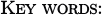Borel probability measures, weak* topology, compactness, payoff functions, reaction correspondences, Nash equilibrium, fixed point theorem.